# 叶池

Leafpool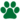独行猫 (Q648)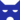影族 (Q628)[临时]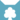天族 (Q632)[临时]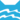河族 (Q630)[临时]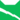雷族 巫医雷族 首席巫医
（继任自炭毛 (Q3157)雷族 猫后雷族 武士雷族 首席巫医影族 首席巫医[临时]
（继任自小云 (Q3318)天族 首席巫医[临时]
（继任自回声之歌 (Q2736)河族 巫医[临时]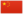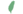小树叶 Leafkit  小葉
 叶爪 Leafpaw  葉掌
 叶池 Leafpool  葉池

Q3383：雷族母猫，自《新预言》系列登场的角色

## 细节

### 外貌

• 她是一只身形娇小:19矫健、:114琥珀色眼睛:144的淡:19棕色虎斑母猫，:猫物表有着白色的胸脯和脚掌。:104

## 语录

—— 叶池的想法，关于她的孩子们，《族群秘事》，第180页

—— 叶池和夜云，在狮焰和风皮打斗时，《暗夜密语》，第17章

—— 叶池对狮焰，《月光印记》，第101页

—— 叶池催促火星吃款冬花梗，在火星得绿咳症时，《暗夜长影》，第137-137页

—— 叶池对蛛足，关于孩子，《暗夜长影》，第206页

—— 叶池对影兆，关于影兆的命运，《迷雾明光》，第278页

## 登场书目

• 午夜追踪 (Q139)[主人公][主要角色][视角人物][首次登场🐱]
• 新月危机 (Q140)[主人公][主要角色][视角人物]
• 重现家园 (Q141)[主人公][主要角色][视角人物]
• 星光指路 (Q142)[主人公][主要角色][视角人物]
• 黄昏战争 (Q143)[主人公][主要角色][视角人物]
• 日落和平 (Q144)[主人公][主要角色][视角人物]
• 预视力量 (Q152)[配角]
• 暗河汹涌 (Q153)[配角]
• 驱逐之战 (Q154)[配角]
• 天蚀遮月 (Q155)[配角]
• 暗夜长影 (Q156)[配角]
• 拂晓之光 (Q157)[视角人物]
• 第四学徒 (Q158)[配角]
• 战声渐近 (Q159)[配角]
• 暗夜密语 (Q160)[配角]
• 月光印记 (Q161)[配角]
• 武士归来 (Q162)[配角]
• 群星之战 (Q163)[配角]
• 学徒探索 (Q170)[配角]
• 雷影交加 (Q171)[配角]
• 天空破碎 (Q172)[配角]
• 极夜无光 (Q173)[配角]
• 烈焰焚河 (Q174)[配角]
• 风暴来袭 (Q175)[配角]
• 迷失群星 (Q3563)[配角]
• 静默冰融 (Q3579)[对话提及]
• 叠影重障 (Q3624)[对话提及]
• 暗由心生 (Q4183)[视角人物]
• 无星之地 (Q4385)[对话提及]
• 迷雾明光 (Q4621)[配角]
• 河翻浪涌 (Q4643)[间接提及]
• 火星的探索 (Q176)[配角]
• 天族的命运 (Q178)[间接提及]
• 黑莓星的风暴 (Q182)[配角]
• 虎心的阴影 (Q185)[对话提及]
• 鸦羽的拷问 (Q186)[配角]
• 松鼠飞的希冀 (Q3561)[主要角色][死亡时刻✝]
• 灰条的誓言 (Q4057)[对话提及]
• 一星的告解 (Q4826)[配角]
• 冬青叶的故事 (Q187)[对话提及]
• 雾星的征兆 (Q188)[对话提及]
• 叶池的祈愿 (Q191)[主人公][主要角色][视角人物]
• 鸽翅的沉默 (Q192)[配角]
• 褐皮的族群 (Q3566)[配角]
• 蛾翅的秘密 (Q3628)[配角]
• 变革之风 (Q4411)[配角]
• 族群救星 (Q214)[配角]
• 武士之心 (Q215)[配角]
• 族群的秘密 (Q199)[追溯修订]
• 族群的猫 (Q200)[配角]
• 族群的守则 (Q201)[视角人物]
• 族群的战争 (Q202)[配角]
• 终极指南 (Q204)[配角]
• 日落之后：正确的选择？ (Q4323)[配角]

## 参考文献

这篇文章基于CC BY-SA 3.0许可使用了猫武士维基（英语）Leafpool一文中的部分内容。
1. 《松鼠飞的希望》第二十四章 (Q3968)
2. 《火星的探索》尾声 (Q1691)
3. 午夜追踪
4. 重现家园
5. Su Susann (2016-09-30). 站内截图. -|Facebook-. 原页面 归档于 2017-10-31. “As I said, Adderfang is a descandant of Spottedpelt and Pinestar is, trough the blood of his father, related to Gorseclaw.”
6. 呼唤野性
7. 族群的猫》，英文
8. 火星的探索
9. 黄昏战争
10. 艾琳·亨特访谈6
11. 松鼠飞的希冀